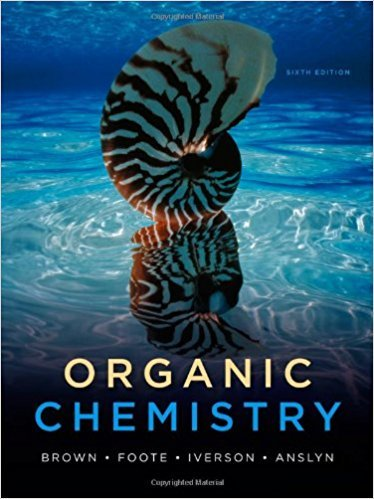×
×

# Following is a 1 H-NMR spectrum of 2-butanol. Explain whyISBN: 9780840054982 88

## Solution for problem 13.7 Chapter 13

Organic Chemistry | 6th Edition

• Textbook Solutions
• 2901 Step-by-step solutions solved by professors and subject experts
• Get 24/7 help from StudySoup virtual teaching assistantsOrganic Chemistry | 6th Edition

4 5 1 410 Reviews
21
0
Problem 13.7

Following is a 1 H-NMR spectrum of 2-butanol. Explain why the CH2 protons appear as a complex multiplet rather than as a simple quintet. 98 654 7 0 a a b b e e c d c 321 ppm Chemical Shift ( ) expansion Solvent OH d c

Step-by-Step Solution:
Step 1 of 3

Molecular Mas and Formula Mass  Molecular mass o Sum of atomic masses of ions in a molecular formula  Unit= amu  Formula mass o Sum of atomic masses of ions in a formula unit Conversion:  Always go to moles and then convert to desired unit Chemical reaction  Bonds are broken and new ones form  Properties of new substance are different...

Step 2 of 3

Step 3 of 3

##### ISBN: 9780840054982

Unlock Textbook Solution